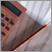1500.0 - A guide for using statistics for evidence based policy, 2010
ARCHIVED ISSUE Released at 11:30 AM (CANBERRA TIME) 20/10/2010  First Issue
Page tools:Print All
Contents >> Understanding statistical concepts and terminologyUNDERSTANDING STATISTICAL CONCEPTS AND TERMINOLOGY

Understanding basic and more advanced statistical concepts and terminology is vital for making effective use of statistical information and for analysing and drawing conclusions from data. This includes an ability to recognise and apply basic statistical concepts such as percentages, measures of spread, ratios and probability. An understanding of more complex statistical concepts is necessary for more in-depth analysis of data. It is also necessary to be able to understand and interpret statistical information presented in tables, graphs and maps.

Qualitative and quantitative research

It is useful to have an understanding of qualitative and quantitative research techniques, and knowing which type of research is appropriate for the issue you are investigating.

Qualitative data are usually text based and can be derived from in-depth interviews, observations, analysis of written documentation or open ended questionnaires. Qualitative research aims to gather an in-depth understanding of human behaviour and the reasons that govern such behaviour. The discipline investigates the why and how of decision making, not just what, where and when. It allows researches to explore the thoughts, feelings, opinions and personal experiences of individuals in some detail, which can help in understanding the complexity of an issue. Smaller but focused samples may be needed rather than large random samples. Qualitative research is also highly useful in policy and evaluation research, where understanding why and how certain outcomes were achieved is as important as establishing what those outcomes were. Qualitative research can yield useful insights about program implementation such as: Were expectations reasonable? Did processes operate as expected? Were key players able to carry out their duties?

Quantitative data are numerical data that can be manipulated using mathematical procedures to produce statistics. Quantitative research is the systematic scientific investigation of quantitative properties, phenomena and their relationships. The objective of quantitative research is to develop and employ statistical models, theories and/ or hypotheses pertaining to phenomena and relationships. The process of measurement is central to quantitative research because it provides the fundamental connection between empirical observation and statistical expression of quantitative relationships.

The following section will focus on quantitative research techniques.

Measures of central location and measures of spread

Measures of central location refers to the ‘centre’ of all observations in a dataset. This will give some idea of the most typical value for a particular set of observations. This centre can be expressed as a mean, median or mode.

Mean: The mean of a set of numeric observations is calculated by summing the values of all observations in a dataset and then dividing by the number of observations. It is also known as the arithmetic mean or the average.

Median: If a set of numeric observations are ordered by value, the median corresponds to the value of the middle observation in the ordered list.

Mode: In a set of data, the mode is the most frequently observed value. A set of data can have more than one mode or no modes. In many datasets, the most frequently observed value will occur around the mean and median values, but this is not necessarily the case, particularly where the distribution of the dataset is uneven.

Measures of spread indicates how values in a dataset are distributed around the central value. Some commonly used measures of spread are the range and standard deviation.

Range: The range represents the actual spread of data. It is the difference between the highest and lowest observed values. As with calculation of the median, it is helpful to order data observations to find the highest and lowest values.

Standard deviation: This measures the scatter in a group of observations. It is a calculated summary of the distance each observation in a data set is from the mean. Standard deviation gives us a good idea whether a set of observations are loosely or tightly clustered around the mean

For more information, see Statistical Language!, 2008 (cat. no. 1332.0.55.002) an online resource available on the ABS website. It is designed to help improve understanding of fundamental statistical concepts and equip users with the basic knowledge required for critical and informed use of data.

Being able to understand and interpret statistical information presented in tables, graphs and maps is an essential skill for data analysis. When reading data in tables, graphs and maps it is often helpful to follow a logical process. The following steps may help you analyse and interpret data in tables, graphs and maps:

• observe the layout in order to understand how the data are arranged. Check the row and column names in a table; the x and y axis in a graph; or the key of the map to get a clear idea of the variables being displayed. Are there just numbers, or are percentages also used?

• next, scan any totals as this may assist you to get an idea of any overall trends in the data

• also, make sure you look at any additional information and footnotes as they may contain important information that can be used to assess the accuracy of the data, and/or to understand the limitations of the data

• now, have a look at the data and how it is represented. Does anything stand out? Are there any trends in the data? Are the data uniform? What relationships can you see between the data? What summary measures could you use to gain a better understanding of the data? What conclusions can be drawn?

 Previous Page Next Page